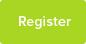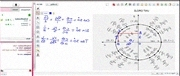Geometric Pogression

Formulae for Geometric pogression

Date : 19/06/2012Author Information

Uploaded by : Sankar
Uploaded on : 19/06/2012
Subject : Maths

Introduction :-

Mean ,one of the kind of averages,is generally defined for a set of data . The mean of data set is sum of the numbers divided by number of data elements.here is a tutoring on online geometric mean .

There are three types of calculation of means of data depending on the sequence of data.

i) Arithmetic Mean

ii)Geometric mean

iii) Harmonic mean

Geometric mean of a progression :-

The geometric mean is calculated for a geometric distribution .The Geometric distribution or progression is the sequence of numbers such that ratio of successive numbers is constant.The general geometric distribution is given as a, ad ,ad2 , . . . . . , ad(n-1). here initial number is "a" and common ratio is "d".

eg: 1,3,9

Here initial number is 1 and common ratio is 3.

The mean "b"of a geometric distribution a,b,c is given by b= [sqrt(a*c)]

Formulae for Geometric progressions or distribution (G.P):-

i) nth term of an G.P is a*r^(n-1) ii) sum to infinite terms of a G.P is a/(1-r) where r is <1 iii) If a,b,c are in geometric progression then b= [sqrt(a*c)] iv) Sum to numbers in G.P is a(1-r^n ) / (1-r)

Example problems on geometric mean :-

Ex 1)Find the geometric mean (G.M)of numbers 2,32

Solution)Geometric mean of two numbers is given by square root (32*2) =square root (64) =8

Hence G.M of two numbers is 2 ,32 is 8

Ex2)Find the geometric mean of geometric sequence 1,3,6,9

Solution)geometric mean of sequence 1,3,6,9 is

sqrt( 1*3*6*9)

=sqrt(162)

=12.72

EX3)Find the geometric mean of distribution 2,4,8

Solution )Geometric mean is given by sqrt( 2*4*8)

=sqrt(64)

=8

This resource was uploaded by: Sankar